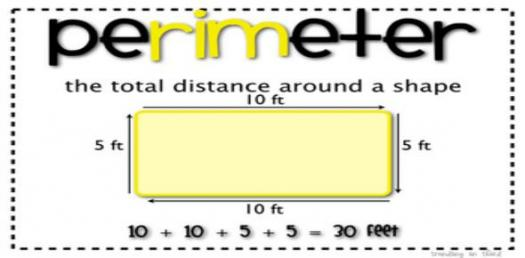10 Questions | Attempts: 679SettingsIn the mathematical context, the perimeter is the line that surrounds a plane figure with two dimensions. Put, it is the measure of the space a shape will occupy. The perimeter of a circular figure is its circumference. What is the perimeter of other two dimensional shapes that you know? Attempt this quiz now!Back to top